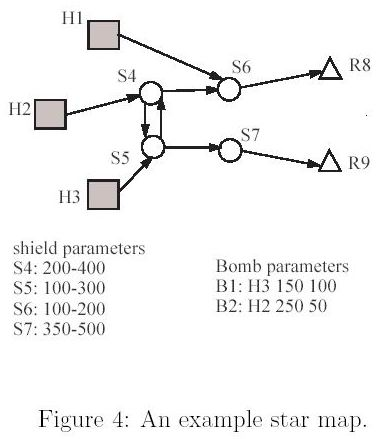Online JudgeProblem SetAuthorsOnline ContestsUser
Web Board
F.A.Qs
Statistical Charts
Problems
Submit Problem
Online Status
Prob.ID:
Register
Authors ranklist
Current Contest
Past Contests
Scheduled Contests
Award Contest
Register

Language:
Space AI Bombs
 Time Limit: 1000MS Memory Limit: 10000K Total Submissions: 331 Accepted: 65

Description

The time is year 3000. Human beings have settled on planets in many solar systems and have a star war with an alien species called Romulans. The human scientists design a new weapon called AI bomb which is capable of space travel across the vast space. Before launching the weapons, humans send probes to collect Romulan's defense parameters. The data shows that Romulans have set up shields in the routes to their home worlds. Fortunately, some secret information reveals that the shield can be penetrated using an ion beam with a particular range of frequency. It is possible to pass the shield if an AI bomb emits an ion beam within that frequency. Now,human scientists have plotted an interstellar map between several human planets and Romulan planets. The map is a directed graph like Figure 4. In the figure, human planets are drawn in boxes (denoted as Hx) and Romulan worlds are drawn in triangles (denoted as Rx), where x is an integer number. A shield is drawn as a circle in the figure (denoted as Sx).
Since humans only know where the shields are but do not know the frequency of each shield, they decide to launch a large number of AI bombs. Each bomb is configured to emit an ion beam at a particular frequency at first. Once an AI bomb passes a shield, it will modulate its frequency to a different value by increasing or decreasing a predefined value. For example, in Figure 4, an AI bomb B1 is launched from H3 with initial frequency 150 and an interval (+/-) 100. So, when B1 penetrates shield S5, it may modulate its frequency to 50, 250, or keep its previous frequency 150. After that, the bomb can choose any routes available in the star map. In the example, the bomb B1 is possible to reach Romulan homeworld R9 by penetrating S5with the original frequency 150 and then passing S4 by changing its frequency to 250 and keeping frequency 250 to pass S5 again and by changing its frequency to 350 in order to penetrate S7 and then finally nuking Romulan planet R9.
Unfortunately, Romulans knows what humans are planning. Their spies got the map and the bomb parameters. Of course, Romulans have shield parameters at hand.They want to know if there are any AI bombs which can reach their homeworlds under current shield settings. Please note that human AI bombs can choose any route to travel. If an AI bomb has any chance to reach a Romulan's home world, then the bomb must be reported.
Please write a program for the Romulan to defend vicious humans. To simplify the problem, we restrict the frequency values between 0 and 1000. When a bomb's new frequency is outside the range, the new frequency is invalid.

Input

The test data begins with a number n in a line which is the number of test cases. In each test case, it begins with two numbers v and e in a line where v is the number of vertices (including human planets, Romulan planets, and shields) and e is the number of directed edges, 2<= v <= 100000 and 2 <= e <= 500000. For convenience,
the vertices are indexed starting with 1.
Next, a line beginning with 'human m' tells that there are m human planets. Following the string are m integers, which are the indices of human planets.
Same as above, a string 'romulan k' is used to tell the vertex indices of Romulan's planets.
A string 'shield x' begins the shield parameters, where x is the number of shields.
Each shield parameter is described by (s l u), where s is the shield's index, l is the lower bound of the range, and u is the upper bound of the range. The values of l and u is between 0 and 1000.
A string 'edge u' begins the directed edge data, where u is the number of edges.Each edge is described by (s d), where s is the index of the source vertex and d is the index of end vertex.
A string 'bomb p' begins with the data of deployed AI bombs, where p is the number of bombs and 1 <= p <= 10000. Each bomb is described by (h f i), where h is the index of a vertex (i.e., a human planets where the bomb is located), f is the initial frequency,and i is the interval to be increased/decreased.

Output

Please output the number of bombs that can possibly reach any of the Romulan homeworlds in one line for each test case. Note that, a bomb may be able to reach more than one Romulan planets. In that case, it is still counted as 1.

Sample Input

```1
9 9
human 3 1 2 3
romulan 2 8 9
shield 4
4 200 400
5 100 300
6 100 200
7 350 500
edge 9
1 6
6 8
2 4
4 6
4 5
5 4
3 5
5 7
7 9
bomb 2
3 150 100
2 250 50```

Sample Output

`2`

Source

[Submit]   [Go Back]   [Status]   [Discuss]Home PageGo BackTo top